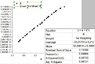# Semi ln plot- uncertainty estimation

• I
• Imperatore

#### Imperatore

Hello there!

There is a problem with calculating the uncertainty from semi- ln plot. The linear fitting gives standard errors as you can see in attached picture. In the Y axis are ln J values, obviously. If the intersection with y-axis, x=0, then we get the point y=b=-33,21, and it's ln J', so the J' equals: exp(-33,21), ok it works fine. But the uncertainty of J' gives then value of exp(0,212), which is about 10^14 factor greater than the calculated value of J'.

What's wrong with my solution?

#### Attachments

•semi ln plot.jpg
49.9 KB · Views: 511
But the uncertainty of J' gives then value of exp(0,212)
That is not what it gives. The uncertainty of 0.212 means your value 33.21 could also be 0.212 larger or smaller (there is a more mathematical statement behind it, but let's keep it at that level). What is exp(33.21 ± 0.212)?

•Imperatore
I got it. Uncertainty is |exp(-33.21-0.212)-exp(-33.21+0.212)| equals 1,6 e-15 Is it really that simple?

It is not that simple, and a better treatment would likely lead to asymmetric uncertainties. But looking at the up and down deviation gives some reasonable approximation.

•Imperatore
Oh, I should devided that relation |exp(-33.21-0.212)-exp(-33.21+0.212)| by 2, obviously.